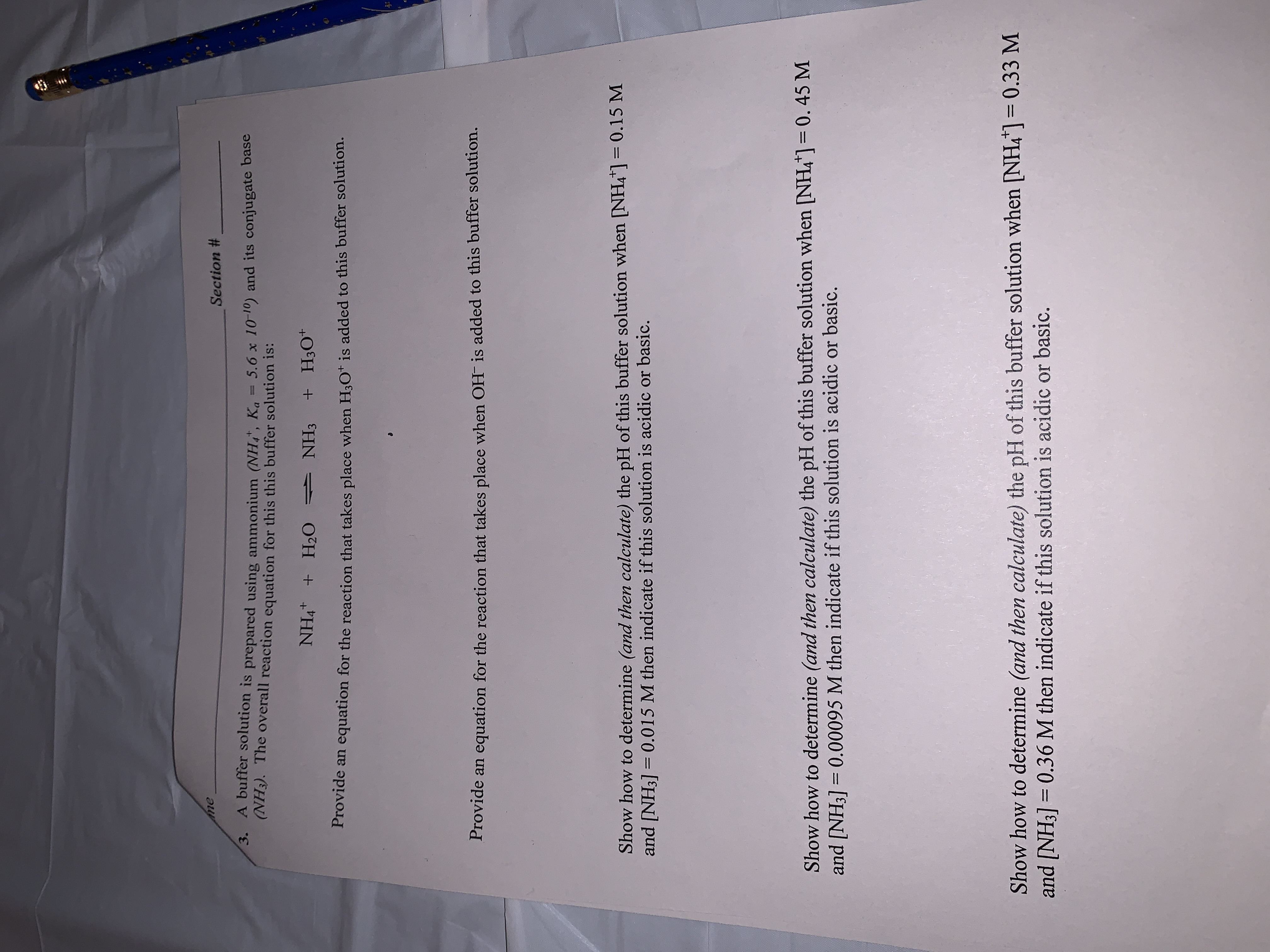# meSection #3. A buffer solution is prepared using ammonium (NH, Ka 5.6 x 10-10) and its conjugate base(NH3). The overall reaction equation for this this buffer solution is:NH4 H2 NH3 +H30Provide an equation for the reaction that takes place when H3O+ is added to this buffer solution.Provide an equation for the reaction that takes place when OH- is added to this buffer solution.Show how to determine (and then calculate) the pH of this buffer solution when [NH4"]and [NH3]= 0.015 M then indicate if this solution is acidic or basic.0.15 MShow how to determine (and then calculate) the pH of this buffer solution when NH4]= 0. 45 Mand [NH3] 0.00095 M then indicate if this solution is acidic or basic.Show how to determine (and then calculate) the pH of this buffer solution when [NH4] = 0.33 Mand [NH3] 0.36 M then indicate if this solution is acidic or basic.

Question
47 views

Chem 1121help_outlineImage Transcriptioncloseme Section # 3. A buffer solution is prepared using ammonium (NH, Ka 5.6 x 10-10) and its conjugate base (NH3). The overall reaction equation for this this buffer solution is: NH4 H2 NH3 +H30 Provide an equation for the reaction that takes place when H3O+ is added to this buffer solution. Provide an equation for the reaction that takes place when OH- is added to this buffer solution. Show how to determine (and then calculate) the pH of this buffer solution when [NH4"] and [NH3]= 0.015 M then indicate if this solution is acidic or basic. 0.15 M Show how to determine (and then calculate) the pH of this buffer solution when NH4]= 0. 45 M and [NH3] 0.00095 M then indicate if this solution is acidic or basic. Show how to determine (and then calculate) the pH of this buffer solution when [NH4] = 0.33 M and [NH3] 0.36 M then indicate if this solution is acidic or basic. fullscreen
check_circle

Step 1

The given reaction:

Step 2

Equation for the reaction when H3O+ is added to the buffer solution:

Step 3

Equation for the reaction when OH- is add...

### Want to see the full answer?

See Solution

#### Want to see this answer and more?

Solutions are written by subject experts who are available 24/7. Questions are typically answered within 1 hour.*

See Solution
*Response times may vary by subject and question.
Tagged in

### Equilibrium Concepts